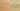ConditionalIsSequence

Misconception:

if (c) A else B is equivalent to if (c) A; if (!c) B.Incorrect

If-else is equivalent to sequence of two ifs

Correct

If-else can behave differently from sequence of two ifs

CorrectionHere is what's right.

An if-else-statement always executes only one of the two bodies:

if (c) {
a();
} else {
b();
}

A sequence of two if-statements, however, may execute both bodies:

if (c) {
a();
}
if (!c) {
b();
}

Specifically, if evaluating c has side-effects, or if the value of c changes between the two tests (if (c) ..., if (!c) ...), then the two are different.

Example Causes for Differences

Condition with side effects:

boolean c() {
System.out.println("c() called"); // side effect
return true;
}

void ifElse() {  // prints once
if (c())  a()
else      b();
}

void twoIfs() {  // prints twice
if (c())  a();
if (!c()) b();
}

Condition depending on state affected by a case:

boolean state;

boolean c() {
return state;  // accesses state that can be different for each call
}

void a() {
state = false;
}

void ifElse() {  // calls a()
state = true;
if (c())  a()
else      b();
}

void twoIfs() {  // calls a() and b()
state = true;
if (c())  a();
if (!c()) b();
}

OriginWhere could this misconception come from?

If students previously learned a functional language, or if they remember functions defined by cases in mathematics, they may see a sequence of conditional statements as a declarative specification of different cases, and not as a sequence of execution steps.

SymptomsHow do you know your students might have this misconception?

This misconception can be detected by asking students to draw a control-flow graph of a sequence of if-statements.

ValueHow can you build on this misconception?

An occurrence of this misconception can be an opportunity for discussing the following aspects:

Functional vs. Imperative Programming

Students with this misconception may think in a more functional and less imperative way: they may see a sequence of if statements as a set of patterns to be matched and not really as a sequence of execution steps.

Nesting of If Statements

The situation where if statements are nested inside else is usually formatted so it looks like a multi-way branch (leaving out the curly braces for the else):

if (a) {
a();
} else if (b) {
b();
} else {
c();
}

This is different from:

if (cond1) {
a();
}
if (!cond1) {
if (cond2) {
b();
}
if (!cond2) {
c();
}
}

Switch Statements

This misconception is related to fallthrough (the absence of breaks) between cases of switch statements: The cases of a switch are only mutually exclusive if a break is placed at the end of each case.

Java